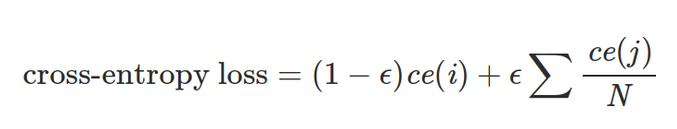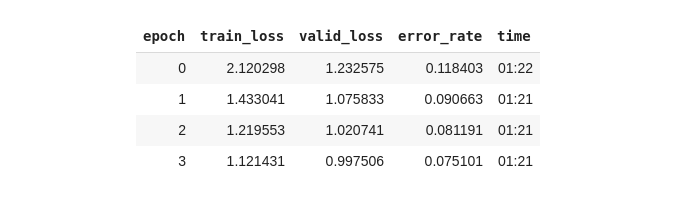# 解决过拟合：如何在PyTorch中使用标签平滑正则化

## 标签平滑？## PyTorch中的使用

``````def linear_combination(x, y, epsilon):
return epsilon*x + (1-epsilon)*y``````

``````import torch.nn.functional as F

def reduce_loss(loss, reduction='mean'):
return loss.mean() if reduction=='mean' else loss.sum() if reduction=='sum' else loss

class LabelSmoothingCrossEntropy(nn.Module):
def __init__(self, epsilon:float=0.1, reduction='mean'):
super().__init__()
self.epsilon = epsilon
self.reduction = reduction

def forward(self, preds, target):
n = preds.size()[-1]
log_preds = F.log_softmax(preds, dim=-1)
loss = reduce_loss(-log_preds.sum(dim=-1), self.reduction)
nll = F.nll_loss(log_preds, target, reduction=self.reduction)
return linear_combination(loss/n, nll, self.epsilon)``````

``````from fastai.vision import *
from fastai.metrics import error_rate

# prepare the data
path = untar_data(URLs.PETS)
path_img = path/'images'
fnames = get_image_files(path_img)

bs = 64
np.random.seed(2)
pat = r'/([^/]+)_\d+.jpg\$'
data = ImageDataBunch.from_name_re(path_img, fnames, pat, ds_tfms=get_transforms(), size=224, bs=bs) \
.normalize(imagenet_stats)

# train the model
learn = cnn_learner(data, models.resnet34, metrics=error_rate)
learn.loss_func = LabelSmoothingCrossEntropy()
learn.fit_one_cycle(4)``````## 结论

Deephub翻译组: 李爱 (Li Ai)

###### 评论